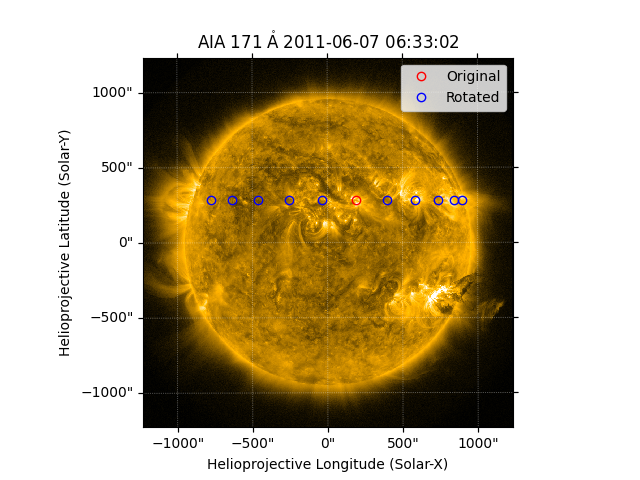# Differentially rotating a coordinate#

How to differentially rotate a coordinate.

The example uses the RotatedSunFrame coordinate metaframe in sunpy.coordinates to apply differential rotation to a coordinate. See Differential rotation using coordinate frames for more details on using RotatedSunFrame.

import matplotlib.pyplot as plt
import numpy as np

import astropy.units as u
from astropy.coordinates import SkyCoord

import sunpy.map
from sunpy.coordinates import RotatedSunFrame
from sunpy.data.sample import AIA_171_IMAGE


First, load an AIA observation and define a coordinate in its coordinate frame (here, helioprojective Cartesian). The appropriate rate of rotation is determined from the heliographic latitude of the coordinate.

aiamap = sunpy.map.Map(AIA_171_IMAGE)
point = SkyCoord(187*u.arcsec, 283*u.arcsec, frame=aiamap.coordinate_frame)


We can differentially rotate this coordinate by using RotatedSunFrame with an array of observation times. Let’s define a daily cadence for +/- five days.

durations = np.concatenate([range(-5, 0), range(1, 6)]) * u.day
diffrot_point = SkyCoord(RotatedSunFrame(base=point, duration=durations))


To see what this coordinate looks like in “real” helioprojective Cartesian coordinates, we can transform it back to the original frame. Since these coordinates are represented in the original frame, they will not account for the changing position of the observer over this same time range.

transformed_diffrot_point = diffrot_point.transform_to(aiamap.coordinate_frame)
print(transformed_diffrot_point)

<SkyCoord (Helioprojective: obstime=2011-06-07T06:33:02.770, rsun=696000.0 km, observer=<HeliographicStonyhurst Coordinate (obstime=2011-06-07T06:33:02.770, rsun=696000.0 km): (lon, lat, radius) in (deg, deg, m)
(-0.00406234, 0.04787238, 1.51846026e+11)>): (Tx, Ty, distance) in (arcsec, arcsec, m)
[(-772.69378072, 282.77545584, 1.51502020e+11),
(-635.25851451, 282.86951972, 1.51373625e+11),
(-459.07089956, 282.9428274 , 1.51273829e+11),
(-254.82447782, 282.99074318, 1.51208725e+11),
( -34.98324566, 283.01021568, 1.51182296e+11),
( 397.53637817, 282.96074958, 1.51249466e+11),
( 583.76348605, 282.89496793, 1.51338955e+11),
( 734.3555842 , 282.80682531, 1.51459158e+11),
( 840.22448988, 282.70185963, 1.51602747e+11),
( 895.06498643, 282.58659113, 1.51760989e+11)]>


Let’s plot the original coordinate and the differentially rotated coordinates on top of the AIA observation.

fig = plt.figure()
aiamap.plot(axes=ax, clip_interval=(1., 99.95)*u.percent)

ax.plot_coord(point, 'ro', fillstyle='none', label='Original')
ax.plot_coord(transformed_diffrot_point, 'bo', fillstyle='none',
label='Rotated')
ax.legend()

plt.show()Total running time of the script: (0 minutes 0.367 seconds)

Gallery generated by Sphinx-Gallery